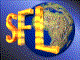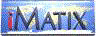| iMatix home page | << | < | > | >>SFLVersion 2.11

### conv_str_number

```#include "sflconv.h"
char *
conv_str_number (
const char *string,                 /*  String to convert                */
int   flags,                        /*  Number field flags               */
char  dec_point,                    /*  Decimal point: '.' or ','        */
int   decimals,                     /*  Number of decimals, or 0         */
int   dec_format,                   /*  How are decimals shown           */
int   width                         /*  Output field width, > 0          */
)
```

#### Synopsis

Converts a string to a number. The number format is defined by one or more of these flags (you add them to get a flags argument):
 FLAG N SIGNED Number is signed. FLAG N DECIMALS Number has decimals. FLAG N ZERO FILL Number has leading zeros. FLAG N THOUSANDS Number has thousands-separators.
The input string may contain digits, decimal point, thousand separators and a sign character or indicator. Formatting characters are only accepted if they correspond to the number format. A blank string is accepted as zero. A space following digits ends the number; anything further is ignored. Returns a string of width digits, including leading sign if that is required. Zeroes are signed with a space. Width must be at least 1. If the flag FLAG_N_DECIMALS is set, the last X digits are decimals, where X is the value of the decimals argument. Decimals are then accepted or rejected depending on the dec_format:
 DECS SHOW ALL Accept decimals. DECS DROP ZEROS Accept decimals. DECS HIDE ALL Reject decimals. DECS SCIENTIFIC Accept decimals.
If the flag FLAG_N_SIGNED is set, accepts a leading or trailing sign, or a financial negative like this: (123). Returns a pointer to the formatted string, or null if the string was rejected. These are the possible reasons for rejection:

• - input number is too large for specified width;
• - input number is signed when no sign is allowed;
• - input number decimals when none are allowed;
• - input number has more decimals than are allowed;
• - more than one sign character in number;
• - malformed financial negative '(123)';
• - more than one decimal point in number;
• - thousand seps when FLAG_N_THOUSANDS is cleared or FLAG_N_ZERO_FILL
• is set (this overrides FLAG_N_THOUSANDS);
• - junk in input string (unrecognised character).

#### Source Code - (sflcvsn.c)

```{
static char
number [FORMAT_MAX + 1];        /*  Cleaned-up return string         */
int
digits,                         /*  Number of digits read so far     */
decs_wanted = decimals;         /*  Number of decimals wanted        */
char
*dest,                           /*  Store formatted number here      */
sign_char,                      /*  Number's sign: ' ', '+', '-'     */
sep_char,                       /*  Thousands separator '.' or ','   */
decs_seen,                      /*  Number of decimals output        */
ch;                             /*  Next character in picture        */
Bool
have_point,                     /*  Have we seen a decimal point     */
have_zero,                      /*  TRUE if number is all zero       */
end_loop;                       /*  Flag to break out of scan loop   */

ASSERT (width <= FORMAT_MAX);
ASSERT (width > 0);
ASSERT (dec_point == '.' || dec_point == ',');

conv_reason = 0;                    /*  No conversion errors so far      */

/*  ---------------------------------   Prepare to copy digits  ---------*/

if ((flags & FLAG_N_THOUSANDS) && !(flags & FLAG_N_ZERO_FILL))
sep_char = dec_point == '.'? ',': '.';
else
sep_char = ' ';                 /*  Reject any thousands separator   */

/*  ---------------------------------   Copy the digits  ----------------*/

digits     = 0;                     /*  No digits loaded yet             */
decs_seen  = 0;                     /*  No decimals output yet           */
sign_char  = ' ';                   /*  Final sign character '+' or '-'  */
end_loop   = FALSE;                 /*  Flag to break out of scan loop   */
have_point = FALSE;                 /*  No decimal point seen            */
have_zero  = TRUE;                  /*  So far, it's zero                */

dest = number;                      /*  Scan through number              */
while (*string)
{
ch = *string++;
switch (ch)
{
case '9':
case '8':
case '7':
case '6':
case '5':
case '4':
case '3':
case '2':
case '1':
have_zero = FALSE;
case '0':
digits++;
*dest++ = ch;
if (have_point)
++decs_seen;
break;

case '-':
case '+':
case '(':
if (sign_char != ' ')
{
conv_reason = CONV_ERR_MULTIPLE_SIGN;
return (NULL);      /*  More than one sign char          */
}
else
if (flags & FLAG_N_SIGNED)
sign_char = ch;
else
{
conv_reason = CONV_ERR_SIGN_REJECTED;
return (NULL);      /*  Number may not be signed         */
}
break;

case ')':
if (sign_char == '(')
sign_char = '-';
else
{
return (NULL);      /*  Malformed financial negative     */
}
break;

case ' ':                   /*  Space ends number after digits   */
end_loop = (digits > 0);
break;

default:
if (ch == dec_point)
{
if (have_point)
{
conv_reason = CONV_ERR_MULTIPLE_POINT;
return (NULL);  /*  More than one decimal point      */
}
else
if (flags & FLAG_N_DECIMALS)
have_point = TRUE;
else
{
conv_reason = CONV_ERR_DECS_REJECTED;
return (NULL);  /*  No decimals are allowed          */
}
}
else
if (ch != sep_char)     /*  We allow sep chars anywhere      */
{
conv_reason = CONV_ERR_INVALID_INPUT;
return (NULL);      /*    else we have junk              */
}
}
if (end_loop)
break;
}

/*  ---------------------------------   Post-format the result  ---------*/

if (flags & FLAG_N_DECIMALS)
{
ASSERT (width > decs_wanted);   /*  At least decimals + 1 digit      */

if (dec_format == DECS_HIDE_ALL)
{
if (have_point)
{
conv_reason = CONV_ERR_DECS_HIDDEN;
return (NULL);          /*  No decimals are allowed          */
}
}
while (decs_seen < decs_wanted) /*  Supply missing decimals          */
{
digits++;
*dest++ = '0';
decs_seen++;
}
if (decs_seen > decs_wanted)
{
conv_reason = CONV_ERR_DECS_OVERFLOW;
return (NULL);              /*  More decimals than allowed       */
}
}
else
decs_wanted = 0;

*dest = 0;                          /*  Terminate the string nicely      */
if (digits > width)
{
conv_reason = CONV_ERR_TOO_MANY_DIGITS;
return (NULL);                  /*  Overflow -- number too large     */
}

if (digits < width)
{
/*  Shift number and null to right of field                          */
memmove (number + (width - digits), number, digits + 1);
memset  (number, '0', width - digits);
}

/*  Store sign if necessary                                              */
if (flags & FLAG_N_SIGNED)
{
ASSERT (width > 1);             /*  At least sign + 1 digit          */
if (number  != '0')
{
conv_reason = CONV_ERR_TOO_MANY_DIGITS;
return (NULL);              /*  Overflow -- no room for sign     */
}
if (sign_char == '(')
{
return (NULL);              /*  Malformed financial negative     */
}
else
if (sign_char == ' ')
sign_char = '+';

if (have_zero)
number  = ' ';           /*  Store sign                       */
else
number  = sign_char;
}

return (number);
}
```

 | << | < | > | >>Copyright © 1996-2000 iMatix Corporation